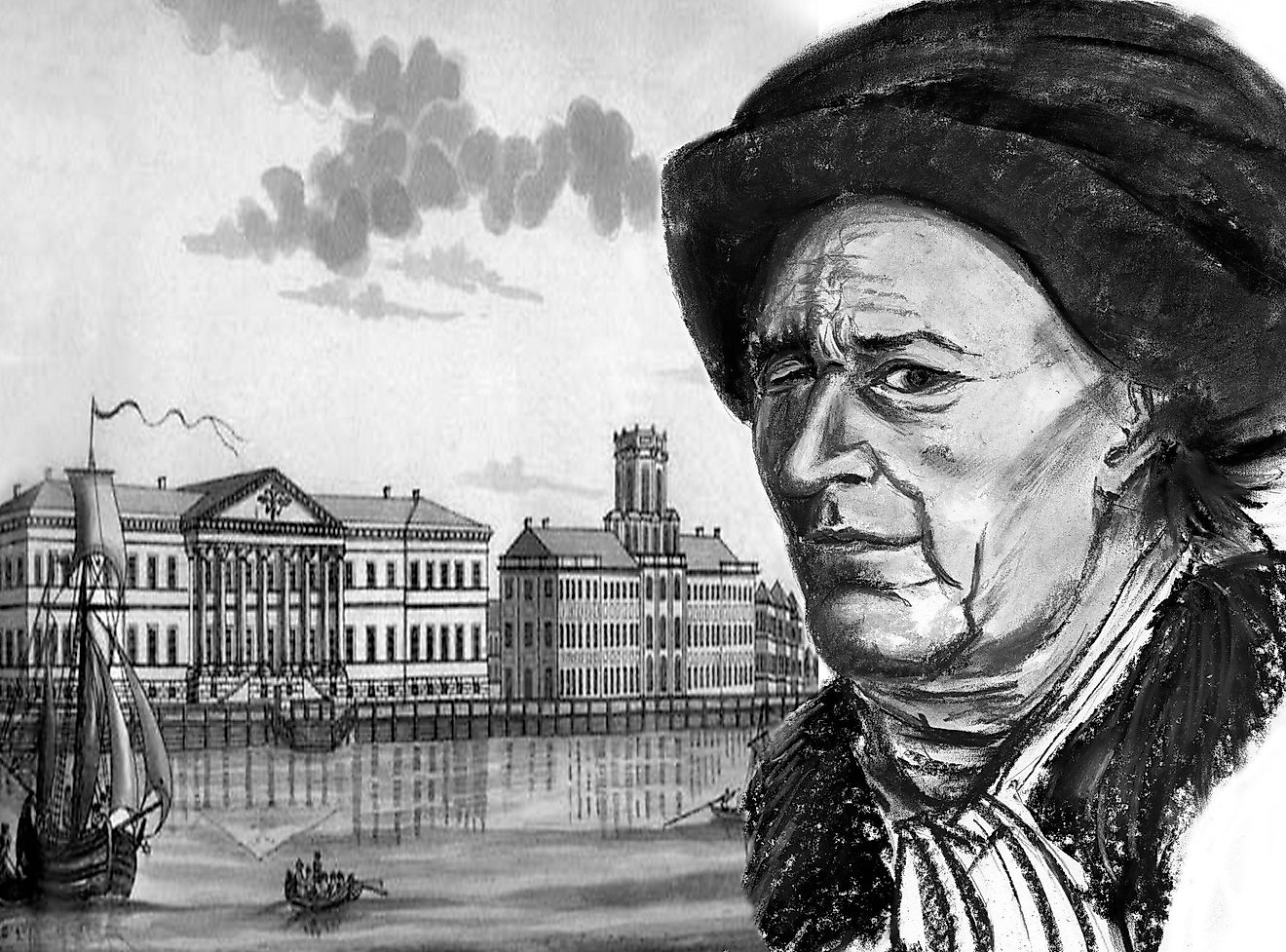# Who Is Known As King Of Mathematics?Leonard Euler is a Swiss, German and Russian mathematician and mechanic who made a fundamental contribution to the development of these sciences.
• Euler was born in 1707 in Basel, Switzerland, and at the age of thirteen, he joined the University of Basel, where he became a Master of Philosophy.
• He has two numbers that are named after him: the Euler's number and the Euler's constant.
• Johann Carl Friedrich Gauss, a German mathematician, is referred to as the

Some hate it, others love it, but all of us are using it in our daily life. Apart from shopping for the best price, figuring out the cost for travel, or understanding sports statistics, mathematics is used in various fields such as medicine, economy, natural and social sciences, etc. It has no universal definition, but many have tried, and some even define it as "what mathematicians do".

However, when it comes to crowning a particular individual as the one who made the most contributions and had the most influence, many agree with one specific person in their mind. To be called a king of such a comprehensive field of study is an incredible privilege. Who could that person be?

## x + Euler = King Of Mathematics

Leonhard Euler, a Swiss mathematician that introduced various modern terminology and mathematical notation, is called the King of mathematics. He was born in 1707 in Basel, Switzerland, and at the age of thirteen, he joined the University of Basel, where he became a Master of Philosophy. Euler is notorious for many critical mathematical discoveries, all of it contained in his circa 92 volumes of work.

This 18th-century mathematician was also a physicist, geographer, astronomer, and a musician. He was responsible for many important discoveries and methods, but mentioning and explaining every single one of them would be impossible for the scope of this article.

However, it should be mentioned that he discovered a new method for quartic equations, proof of Fermat's theorems (another influential 17th-century mathematician), the discovery of more than 60 amicable numbers, the Euler-Langrage equation (an equation that proved the infinitude of prime numbers), development of various tools for real-life application of calculus, etc.

Due to his enormous body of work, documented in his textbooks, he popularized different mathematical notations such as letter i to denote the imaginary unit, the notation for the trigonometric functions, Euler's number, the Greek letter Σ for summations, and many other notations. He even has two numbers that are named after him (and he is the only one); the Euler's number and the Euler's constant!

## Who Is Know As Prince Of Mathematics

There is one more name that is frequently mentioned among mathematicians and physicists when considering groundbreaking work and influence in those fields of study. It is Johann Carl Friedrich Gauss, a German mathematician, sometimes considered to be the "greatest mathematician since antiquity. " Because of his many ideas and seminal works, he is also referred to as the "prince of mathematics".

Considered to be a child prodigy, Gauss finished his doctoral dissertation at the age of 21 and managed to prove the fundamental theorem of algebra, the law of quadratic reciprocity, and published one of the most influential books in all of mathematics, called "Disquisitiones Arithmeticae". Similar to Leonhard Euler, his accomplishments were not only in mathematics, as he was also interested in theoretical astronomy. He invented the electric telegraph and the heliotrope. Not bad for a prince of mathematics!

Share